Pattern Test (x_?Positive), (y_Real?Positive)

PatternTest is closely related to Condition.  PatternTest is used to specify that a certain pattern must meet a  certain condition.  So for example in the next cell (f) is defined when given  an argument that is a positive number.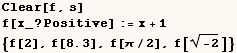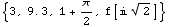In the next example (f) is only defined when given an argument that is a
positive integer.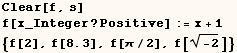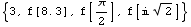In the next example (f) is defined when given an argument that is a positive
integer or a positive real number.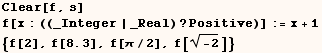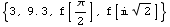In some cases we want to use a test that isn't a built-in unary operator  (Positive, NumericQ, AtomQ, ...).  In that case we can use a pure function as in the next example.  In the next example (f) is only defined when  given an argument that is an integer between 0 and 10.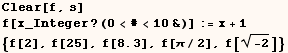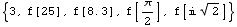In the next cell (f) is only defined when given an argument of any type
between 0 and 10.  Can you see why we need the part about (Im[#]===0) ?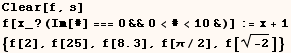We can't use pattern variables in the test portion of (Pattn/;Test), and that
is why the definition in the next cell isn't used.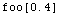Created by Mathematica  (May 16, 2004)

Back to Ted’s Tricks index page